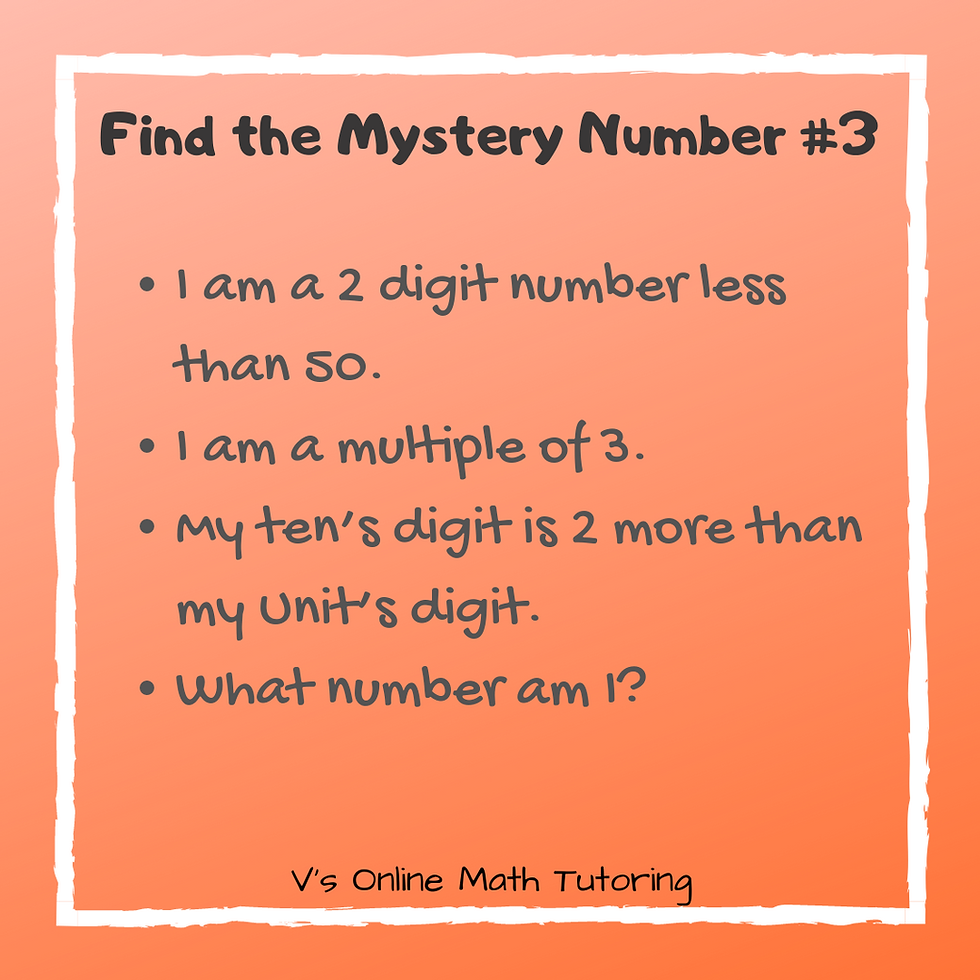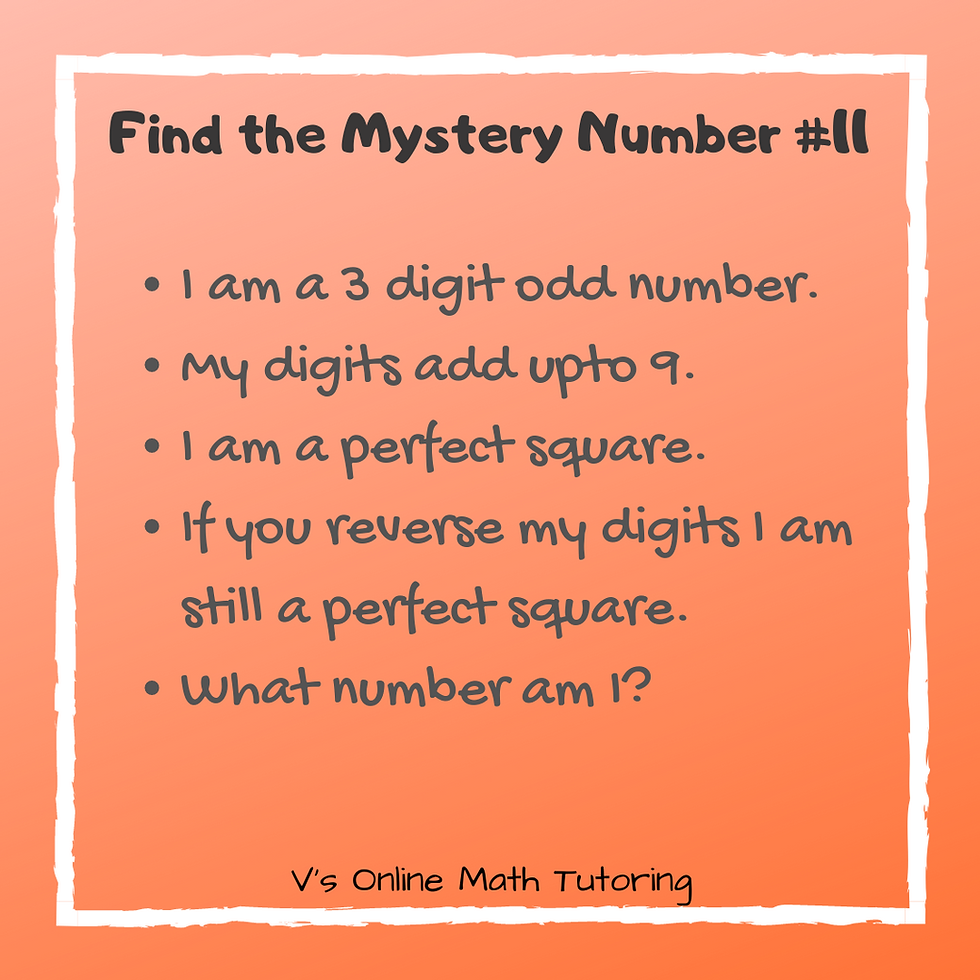top of pageSearch

# "Find the Mystery Number" and Why I Absolutely Love it

Updated: May 11, 2021Find the Mystery number has always been a big hit among my students. My own kid loves it and keeps asking for more. This has been a great way to entertain him and his cousins while we were on road trips and at home. They are also popular in math competitions like MOEMS and Math Kangaroo. This post talks about the various ways you can frame questions with it and how it can help your kids as well.

### 1. Promote growth mindset in kids.

Growth mindset is extremely important in kids. If they are willing to think and learn, they can master anything. It helps them in their adult life too.

Mystery number questions are open ended questions to make them think. Kids learn to make a list, eliminate some responses, and pick the right answer based on the criteria given.

Eg. i) I am a number between 2000 and 8000

My hundreds digit is 6 less than my thousand's digit.

My ten's digit is 6 times my one's digit.

I have no zeros.

What number am I?### 2. Practice a variety of topics

A lot of concepts can be practised through Mystery number puzzles. Place value, divisibility rules, multiples, least common multiples etc.

Eg. i) Practicing place value with Find the Mystery Number

I am a 4 digit number.

My hundred's digit is 4 less than my thousand's digit.

My unit's digit is 7 less than my ten's digit.

My ten's digit is 3 more than my hundred's digit.

My thousand's digit is the largest single-digit number.

What number am I?I've jumbled the order on purpose. I want the kids to figure out what to solve first. Maybe when they start you can give the clues in order.

Eg ii) Practicing divisibility rules and list-making

I am a 2 digit number less than 50.

I am a multiple of 3.

My ten’s digit is 2 more than my Unit’s digit.

What number am I?Eg iii) Practicing LCM and multiples

I am a 2 digit number.

I am a multiple of 4 and 7.

My ten's digit is twice my unit's digit.

What number am I?### 3. The word Mystery gets them excited

Of course throw in the word Mystery and the kids get excited. Take an example of this.

Eg 1) This is so much more fun than asking the kids to just list all the numbers that add upto 10.

I am a 2-digit number.

My tens digit is 2 more than my Unit's digit.

What number am I?This question would require them to make a list but in an indirect way and has the fun element to it.

If I ask my kid to make a list of all numbers that add up to 10, he most certainly will crib about it. But this way he does it happily.

### 4. These can be adapted to almost any age.

From Grade 1 to Grade 8. For the younger kids, they can learn place value. For the Middle and Upper Elementary, they can start their list-making and logical reasoning skills.

Eg i) For Grades 1 and 2 This is how I started my 5-year-old on Mystery Number as well.

I am a 2 digit number.

My ten's digit is 7+2.

My unit's digit is 7-2.

What number am I?It helps them practice both place value and simple single-digit addition and subtraction. A small change to the same worksheets that they do every day.

Eg ii) For grades 1 and 2 The same thing now with 3 digit numbers

I am a 3 digit number.

My hundred's digit is 2 more than my ten's digit.

My ten's digit is 3 less than my unit's digit.

My unit's digit is 9-5.

What number am I?Eg iii) For grades 2 and 3 Starting to make a list

I am a 2 digit number.

My ten's digit is 3 more than my unit's digit.

The sum of my digits is 11.

What number am I?My Kindergartener was able to do these after a couple of days of practising the previous types of questions. Now he can do 3 digit numbers in his head too! A great time pass when we travel :)

Eg iv) For grades 3 and 4 Simple list-making and reasoning

I am a 3 digit number

My digits add up to 14.

My ten's digit is the same as my hundred's digit.

My unit's digit is 2 more than my ten's digit.

What number am I?In this, the kids have to make a list of all number with the hundred's and ten's digit the same and unit's digit being 2 more. Then they pick out the one whose sum is 14.

Of course, after they do a little bit they'll realise that they don't have to make a list. They can just guess one of the digits and calculate the others and add them up to see what works. That's what my kid does.

Eg v) For grades 4 and 5 Fun with palindromes

I am a 4 digit number.

I am a palindrome.

My ten’s digit is 3 more than my thousand’s digit.

The sum of my digits is 22.

What number am I?Eg vi) For grades 6-8 Yes, we can deal with perfect squares too :)

I am a 3 digit odd number

My digits add up to 9.

I am a perfect square.

If you reverse my digits I am still a perfect square.

What number am I?Of course, the grades are just general guidelines. My first grader has solved almost all of these problems. So they can be done by much younger grades. Some older kids might find this challenging as well. Can you solve these Mystery number puzzles?

To summarise, Find the Mystery number puzzles promote a growth mindset and create interest in problem-solving. It can also be used to practice a variety of topics in a fun way and can be adapted to almost any grade level.

For more such problems and other puzzles you can visit my Interesting Math Problems pageDid you enjoy these puzzles? Have a friend or a family member who'll benefit from these as well? Please do share the blog :)